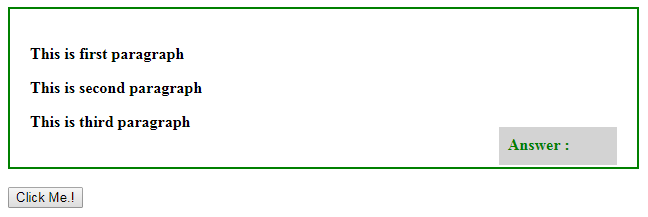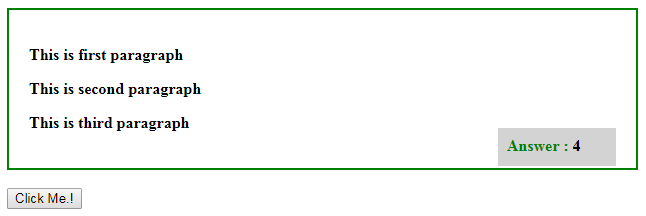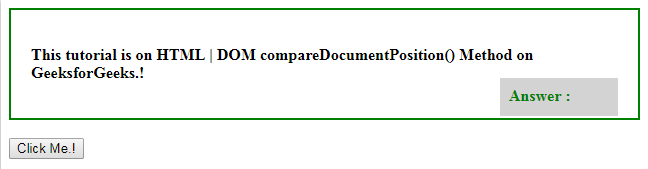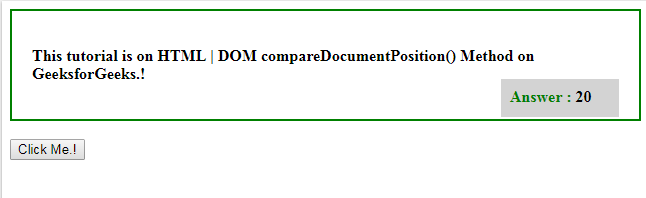# HTML | DOM compareDocumentPosition() Method

The DOM compareDocumentPosition() method is used to compare two nodes and it returns an integer describing where they are positioned in the document.

Syntax:

```node1.compareDocumentPosition(node2)
```

Return Value : This return a integer value and their meaning as follows :

• 1: This means that the two nodes do not belong to the same document.
• 2: This means that the two nodes node1 is positioned after node2.
• 4: This means that the two nodes node1 is positioned before node2.
• 8: This means that the two nodes node1 is positioned inside node2.
• 16: This means that the two nodes node2 is positioned inside node1.
• 32: This means that the two nodes have no relationship or they are two attributes on the same element.

Example-1: This will return only single value.

 ` ` `<``html``> ` `<``style``> ` `    ``div { ` `        ``width: 90%; ` `        ``height: 60%; ` `        ``padding: 20px; ` `        ``border: 2px solid green; ` `        ``font-weight: bold; ` `    ``} ` `     `  `    ``#ans { ` `        ``background-color: lightgrey; ` `        ``display: block; ` `        ``width: 100px; ` `        ``font-weight: bold; ` `        ``height: 20px; ` `        ``padding: 9px; ` `        ``color: green; ` `        ``float: right; ` `        ``margin-top: -20px; ` `    ``} ` `     `  `    ``#res { ` `        ``color: black; ` `    ``} ` ` ` ` `  `<``body``> ` `    ``<``div``> ` `        ``<``p` `id``=``"p1"``> ` `          ``This is first paragraph ` `        `` ` `       `  `        ``<``p` `id``=``"p2"``> ` `          ``This is second paragraph ` `        `` ` `       `  `        ``<``p` `id``=``"p3"``> ` `          ``This is third paragraph ` `        `` ` `       `  `        ``<``p` `id``=``"ans"``>Answer : <``span` `id``=``"res"``> ` `    `` ` `    ``<``br``> ` `   `  `    ``<``input` `type` `= ``button`  `           ``onclick``=``"myFunction()"` `value` `=  ` `           ``"Click Me.!"` `/> ` `    ``<``br``> ` ` `  `    ``<``script``> ` `        ``function myFunction() { ` `            ``var x = p1.compareDocumentPosition(p2); ` `            ``document.getElementById("res").innerHTML = x; ` `        ``} ` `    `` ` ` `  ` ` ` `  ` `

Output:
Before click on the Button:After click on the button:Example-2: This will return combination of two values.

 ` ` `<``html``> ` `<``style``> ` `    ``div { ` `        ``width: 90%; ` `        ``height: 60%; ` `        ``padding: 20px; ` `        ``border: 2px solid green; ` `        ``font-weight: bold; ` `    ``} ` `     `  `    ``#ans { ` `        ``background-color: lightgrey; ` `        ``display: block; ` `        ``width: 100px; ` `        ``font-weight: bold; ` `        ``height: 20px; ` `        ``padding: 9px; ` `        ``color: green; ` `        ``float: right; ` `        ``margin-top: -20px; ` `    ``} ` `     `  `    ``#res { ` `        ``color: black; ` `    ``} ` ` ` ` `  `<``body``> ` `    ``<``div``> ` `       `  `        ``<``p` `id``=``"p1"``>This tutorial is on  ` `          ``<``span` `id``=``"p2"``> ` `             ``HTML | DOM compareDocumentPosition() Method ` `          `` on GeeksforGeeks.! ` `        `` ` `       `  `        ``<``p` `id``=``"ans"``> ` `          ``Answer :  ` `          ``<``span` `id``=``"res"``> ` `        `` ` `       `  `    `` ` `    ``<``br``> ` `   `  `    ``<``input` `type``=``button` `onclick``=``"myFunction()"`  `           ``value``=``"Click Me.!"` `/> ` `    ``<``br``> ` ` `  `    ``<``script``> ` `        ``function myFunction() { ` `            ``var x = p1.compareDocumentPosition(p2); ` `            ``document.getElementById("res").innerHTML = x; ` `        ``} ` `    `` ` ` `  ` ` ` `  ` `

Output:
Before Click on the Button:After Click on the Button: Answer will be 20. ‘4’ means that first node is positioned before the second node and ’16’ second node is positioned inside the first node.Note: The return value can be a combination of values. i.e. if the return value is 20 that means p2 is inside p1, ’16’ and p1 is positioned before p2 ‘4’.

Supported Browsers:

• Google Chorme
• Internet Explorer
• Firefox
• Opera
• Safari

My Personal Notes arrow_drop_upCheck out this Author's contributed articles.

If you like GeeksforGeeks and would like to contribute, you can also write an article using contribute.geeksforgeeks.org or mail your article to contribute@geeksforgeeks.org. See your article appearing on the GeeksforGeeks main page and help other Geeks.

Please Improve this article if you find anything incorrect by clicking on the "Improve Article" button below.

Article Tags :
Practice Tags :

Be the First to upvote.

Please write to us at contribute@geeksforgeeks.org to report any issue with the above content.